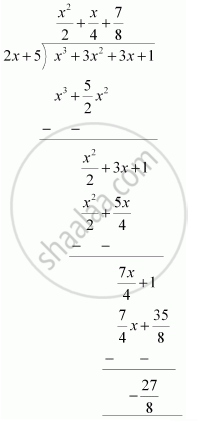# Find the remainder when x^3 + 3x^2 + 3x + 1 is divided by 5 + 2x. - Mathematics

Find the remainder when x3 + 3x2 + 3x + 1 is divided by 5 + 2x.

#### Solution 1

Let p(x) = x3 + 3x2 + 3x + 1

5 + 2x = 0

⇒ 2x = -5

⇒ x = -5/2

therefore "Remainder "= (-5/2)^3 + 3(-5/2)^2 + 3(-5/2) + 1

= -125/8+75/4-15/2+1

= -27/8

Therefore, the remainder is -27/8" ."

#### Solution 2

By long division,Therefore, the remainder is -27/8" ."

Concept: Remainder Theorem
Is there an error in this question or solution?

#### APPEARS IN

NCERT Class 9 Maths
Chapter 2 Polynomials
Exercise 2.3 | Q 1.5 | Page 40